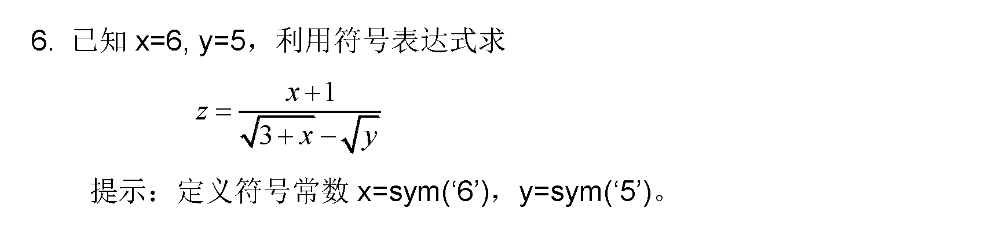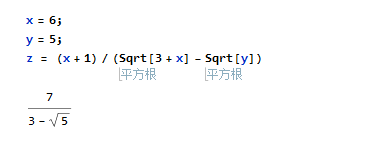# Mathematica入门习题–简单的赋值

• A+

## 第二十二题``````x = 6;
y = 5;
z = (x + 1)/(Sqrt[3 + x] - Sqrt[y])``````• 微信公众号
• 关注微信公众号
•• QQ群
• 我们的QQ群号
•